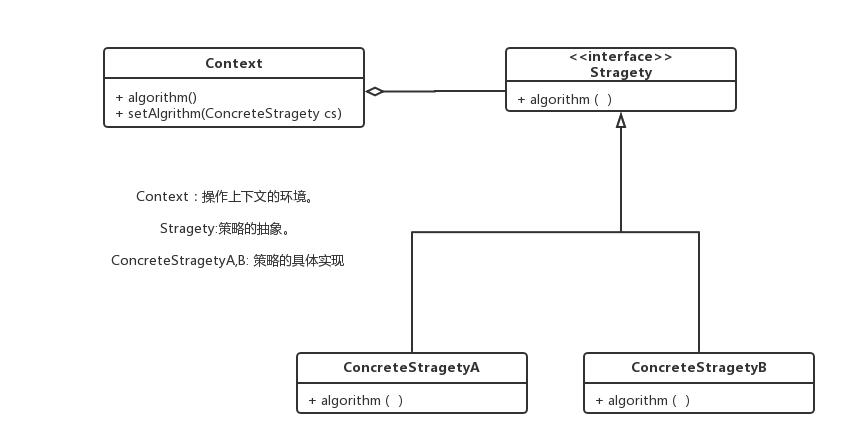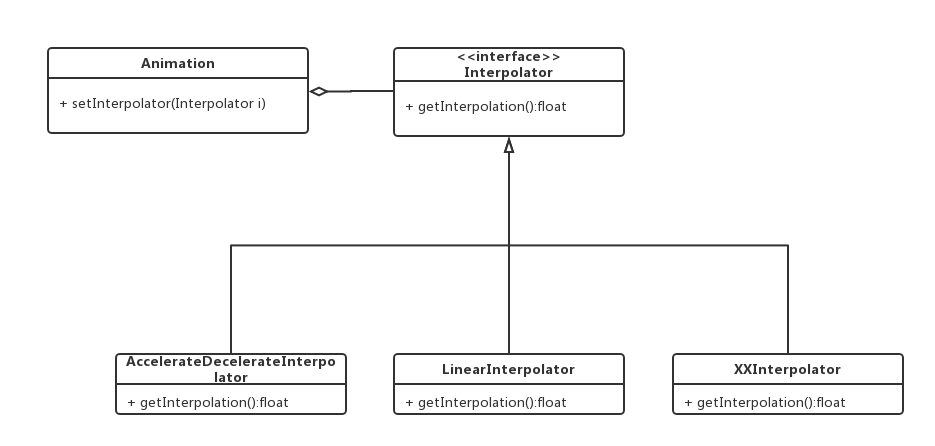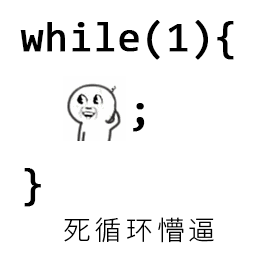# 使用场景

• 针对同一类型的问题有多重解决方式，仅仅是具体行为有差异时。让系统可以动态的选择算法策略。
• 需要安全的封装多重同一类对象时，调用者不会知道算法策略的具体过程。
• 一个类有多个子类，并且在调用的时候用if或switch判断的时候。

# UML# 简单实现

## 先看一下原来的写法

public class PriceCaculator {
public static final int BUS = 1;
public static final int SUBWAY = 2;
public static final int TAXI =3;

private int busPrice(int km){
int busP = 2;
if (km>5)
busP = busP+km-5;
return busP;
}

private int subwayPrice(int km){
int subwayP=0;
if (km<5)
subwayP = 3;
if (km>5&&km<=10)
subwayP=4;
if (km>10)
subwayP=5;
return subwayP;
}

private int taxiPrice(int km) {
return  2 * km;
}

public int getPrice(int km,int type){
if (type==BUS)
return busPrice(km);
if (type == SUBWAY)
return subwayPrice(km);
if (type==TAXI)
return taxiPrice(km);
return 0;
}
}


public class Main {
public static void main(String[] args) {
PriceCaculator priceCaculator = new PriceCaculator();
int price = priceCaculator.getPrice(10,2);
System.out.println(price);
}
}

## 使用策略模式

public interface CalculateStrategy {
int calculatePrice(int km);
}

public class BusCalculate implements CalculateStrategy {
@Override
public int calculatePrice(int km) {
int busP = 2;
if (km>5)
busP = busP+km-5;
return busP;
}
}
public class SubwayCalculate implements CalculateStrategy {
@Override
public int calculatePrice(int km) {
int subwayP=0;
if (km<5)
subwayP = 3;
if (km>5&&km<=10)
subwayP=4;
if (km>10)
subwayP=5;
return subwayP;
}
}
public class TaxiCalculate implements CalculateStrategy {
@Override
public int calculatePrice(int km) {
return  2 * km;
}
}

public class PriceCalculate2 {
private  CalculateStrategy calculateStrategy;
public void setCalculateStrategy(CalculateStrategy calculateStrategy){
this.calculateStrategy=calculateStrategy;
}

public int getPrice(int km){
return calculateStrategy.calculatePrice(km);
}
}

public class Main {
public static void main(String[] args) {
PriceCalculate2 priceCalculate2 = new PriceCalculate2();
priceCalculate2.setCalculateStrategy(new SubwayCalculate());
int price = priceCalculate2.getPrice(10);
System.out.println(price);
}
}

# Android源码中的粗略模式

Animation animation = new AlphaAnimation(1,0);
animation.setInterpolator(new AccelerateDecelerateInterpolator());
imageView.setAnimation(animation);
animation.start();

animation.setInterpolator()其实用的就是策略模式，Android中有很多不同的插值器，都实现了Interpolator接口。

public void setInterpolator(Interpolator i) {
mInterpolator = i;
}

protected void ensureInterpolator() {
if (mInterpolator == null) {
//默认就是加速度插值器,在Animation初始化的时候执行。
mInterpolator = new AccelerateDecelerateInterpolator();
}
}

public boolean getTransformation(long currentTime, Transformation outTransformation) {
......
if ((normalizedTime >= 0.0f || mFillBefore) && (normalizedTime <= 1.0f || mFillAfter)) {
......
//通过策略模式来获取不同的值。
final float interpolatedTime = mInterpolator.getInterpolation(normalizedTime);
applyTransformation(interpolatedTime, outTransformation);
}

......
}# 总结

## 优点

• 结构清晰明了，消除了代码中的一大串选择语句，而是分离为一个个独立的类，代码更清晰。
• 耦合度低，都是基于接口调用和实现，便于拓展和修改。
• 封装更彻底，数据更安全。

## 缺点

• 首先是会产生很多策略类，增加了系统开销。
• 用户要知道所有的策略类。